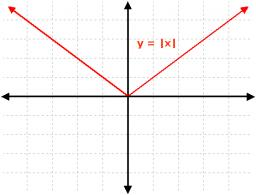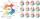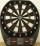# Null points

Calculate the roots of the equation:

$3\mathrm{\mid }x+4\mathrm{\mid }+3\mathrm{\mid }x+5\mathrm{\mid }+2\mathrm{\mid }x+4\mathrm{\mid }=30$

number of roots:  2
x1 =  -8.13
x2 =  -0.63

### Step-by-step explanation:Did you find an error or inaccuracy? Feel free to write us. Thank you!Tips to related online calculators
Do you have a linear equation or system of equations and looking for its solution? Or do you have a quadratic equation?

## Related math problems and questions:

• Eq2 equationsFor each of the following problems, determine the roots of the equation. Given the roots, sketch the graph and explain how your sketch matches the roots given and the form of the equation: g(x)=36x2-12x+5 h(x)=x2-4x+20 f(x)=4x2-24x+45 p(x)=9x2-36x+40
• RootsDetermine the quadratic equation absolute coefficient q, that the equation has a real double root and the root x calculate: 5x 2 +9x + q = 0
• AP 6Calculate the first five items of an arithmetic sequence if it is given: a2 – a3 + a5 = 20 a1 + a6 = 38
• ContestantsIn the competition it was possible to get 0-5 points. In fact, 5 contestants of the 15 best contestants has earned 5 points or 4 points (which have won 10 contestants). The number of contestants who earned 3 points was the same as the number of contestant
• Three points 2The three points A(3, 8), B(6, 2) and C(10, 2). The point D is such that the line DA is perpendicular to AB, and DC is parallel to AB. Calculate the coordinates of D.
• Math testObelix filled a mathematical test in which he answered 25 questions. For every correct answer, he received 5 points, for each bad answer he had 3 points deducted. Obelix gained 36% of all points in the test. How many questions did he solve correctly?
• TrainsFrom station 130 km away started passenger train and after 2.2 hours after the express train, which travels 37 km an hour more. Express train finish journey 7 minutes early. Calculate the average speed of this two trains.
• Equation with absSolve this equation with absolute value member: |3(3x-1)|=8
• TargetPeter, Martin and Jirka were fire in a special target, which had only three fields with values of 12, 18 and 30 points. All boys were firing with the same number of arrows and all the arrows hit the target, and the results of every two boys differed in on
• Balloon and bridgeFrom the balloon, which is 92 m above the bridge, one end of the bridge is seen at a depth angle of 37° and the second end at depth angle 30° 30 '. Calculate the length of the bridge.
• TestingStudents of high school have 10 points for each good solved task. The wrong answer is deducted by 5 points. After solving 20 tasks, student Michael had 80 points. How many tasks did he solve correctly and how many wrong?
• Calculate 6Calculate the distance of a point A[0, 2] from a line passing through points B[9, 5] and C[1, -1].
• Geometric seqFind the third member of geometric progression if a1 + a2 = 36 and a1 + a3 = 90. Calculate its quotient.
• Find d 2Find d in an A. P. whose 5th term is 18 and 39th term is 120.
• TV competitionIn the competition, 10 contestants answer five questions, one question per round. Anyone who answers correctly will receive as many points as the number of competitors answered incorrectly in that round. After the contest, one of the contestants said: We
• Three pointsThree points A (-3;-5) B (9;-10) and C (2;k) . AB=AC What is value of k?Divide number 135 into two additions so that one adds 30 more than 2/5 of the other add. Write the bigger one.